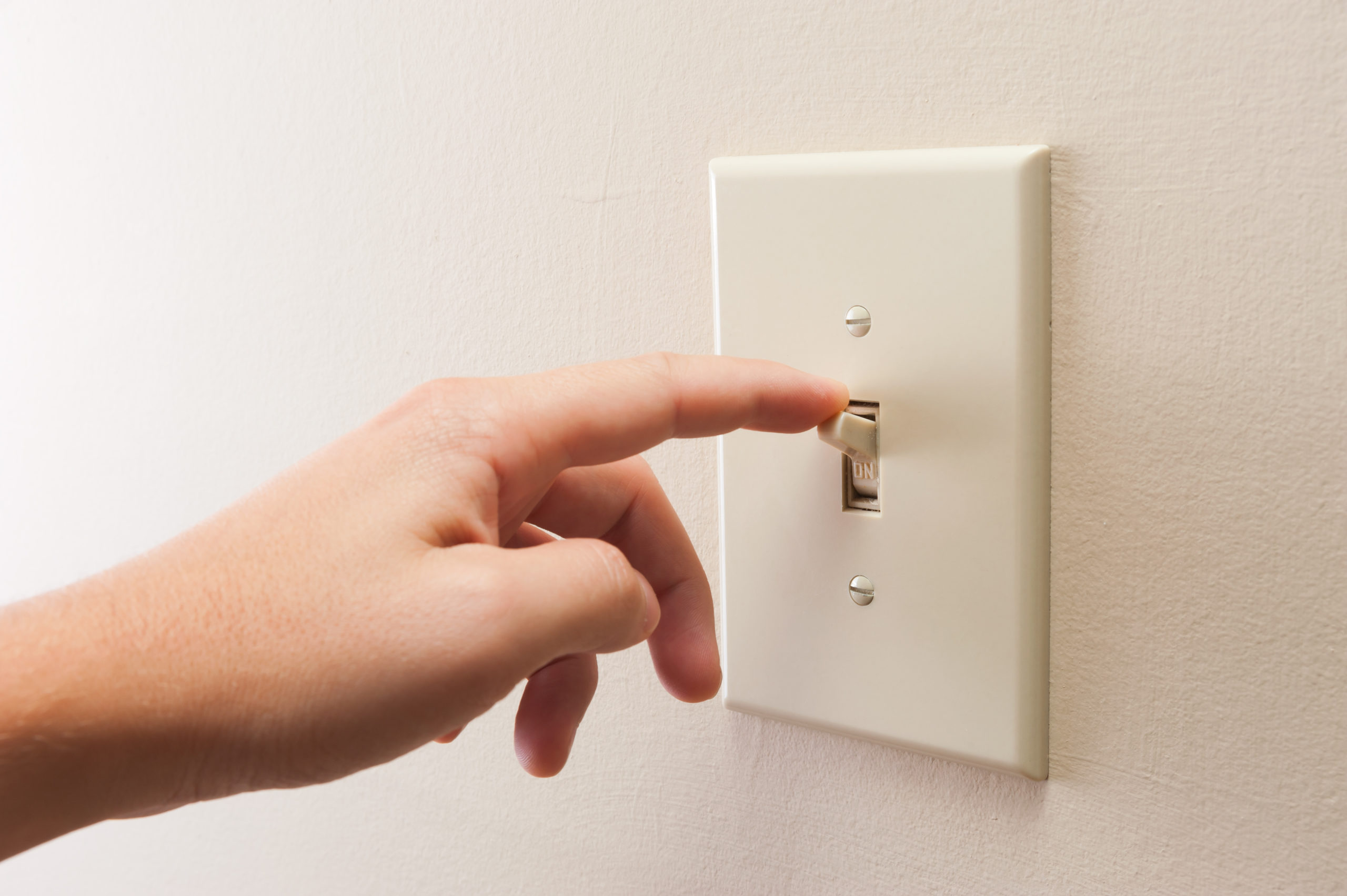## Calculating Electricity Consumption

### Wednesday, August 9, 2017Understanding how items in your home impact your bottom line is an essential step in controlling utility usage. Calculating a particular device or appliance’s consumption is a quick process that can provide invaluable insight.

# 1) Calculate Watts Per Day

First, multiply the unit’s wattage by the number of hours you use it to find the number of watt-hours consumed each day.
For example, let’s say you use a 125 watt television for three hours per day. Your wattage consumption will be 375 watt-hours per day.

# 2) Convert to Kilowatts

Electricity is measured in kilowatt hours (kWh) on your electricity statement, so you’ll have to do some conversions to make sense of the consumption. Since we know that 1 kilowatt is equal to 1,000 watts, you can divide the watt hours per day by 1000 to find out kWh.
375 watt-hours per day / 1000 = 0.375 kWh per day

# 3) Determine Monthly Usage

Electricity is billed in periods, typically 30 day periods.  To find out monthly consumption, you need to multiply daily kWh by the length of the period.
In our example, we’ll assume the billing period is 30 days.
375 watt-hours per day X 30 days = 11.25 kWh per month